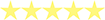# Common Knowledge

## What are your Margin Call and Stop out Levels?

A Margin Call is literally a warning received in your MT4 trading platform to notify you that your account has slipped past a certain percent of the required margin and there is not enough equity (unused balance + floating profits – floating losses) on the account to support your Open trades any further. A Stop Out level is also a certain required margin level in percent, at which the MT4 trading platform will start to automatically close trading positions (starting from the least profitable position and until the margin level requirement is met) in order to prevent further losses into negative territory – below 0 USD.

EXAMPLE: Let’s assume you have an open position of 1 lot on USDCHF in a Classic account with a leverage of 200. The margin will be: 100000/200=500 USD. As we stated earlier, the margin call on Classic accounts occurs when your equity is at 25% of the margin. Therefore, we multiply our margin 500 by 25%. This gives us 125 USD. Furthermore, if your account equity continues to fall further and eventually reaches the point where it becomes equal to 15% of the required margin, your trade will be compulsory closed (stopped out). Following our example, this will happen when your equity is 500*15%=75USD.

## Margin calculation

Here is the basic formula used to calculate margin: Margin=Trade Volume/Account Leverage. Please note that Trade Volume is calculated depending on the currency of your account. While calculating a trade volume, you need to take the Base currency into account. The Base Currency is the first in a currency pair.

EXAMPLE 1. Let’s assume you have a trading account in USD with a leverage of 1:50, and you are going to open a 0.2 lot trade on USDCHF currency pair. The required margin will be: Margin = Trade Volume/Account leverage=20 000 (0.2 lot)/50(our leverage)=400USD.

EXAMPLE 2. Now let’s assume you have a trading account in USD with a leverage of 1:200 and you are going to buy 0.5 lot of the EURUSD currency pair. Given that the current EURUSD exchange rate is 1.3200, the required margin will be: Margin=Trading Volume/Account Leverage=50 000*1.32/200=330USD. In this example we have bought 0.5 lots of EUR, but our account is in USD, that is why we have converted the amount of our trade back to USD – according to the current exchange rate.

## ECN commission

Please note that the calculation of the ECN commission is based upon the volume in USD. All trades on ECN accounts are subject to a commission of 3.5 USD per notional trading amount of 100,000 USD. In other words, the total amount of commission for a round-turn lot will be 7 USD.

EXAMPLE 1. Let’s assume you are going to execute a trade of 1 lot size on USDCHF. Considering that 1 lot on USDCHF is equal to 100,000 USD (which is equal to one Standard Lot), the commission will be 7 USD: ECN commission=7*Trade Volume/100 000=7*100 000/100 000=7 USD.

EXAMPLE 2. A trade of 0.5 lot on EURUSD at the exchange rate of 1.3200 will be subject to a commission of 4.62 USD. Since our base currency is EUR and not USD, we will need to convert it to USD by multiplying our lot size by the current exchange rate: ECN Commission=7*Trade Volume in USD/100 000=7*50 000*1.32/100 000 = 4.62 USD. In the example above EUR is the base currency, so 50,000 EUR will be equal to 50,000*1.32=66,000 USD.